# Heat Flux applied on pipe wall

Hello

I am trying to run a simple model that consists of an extruded circle (forming a cylinder).

The set up is such that a fluid flows through the interior of the cylinder/pipe and there is a heat flux applied on the wall. However, when I read the results for the Nusselt Number, I get incorrect values (compared to literature). When I set heat flux to 0 and set a higher temperature at the wall to simulate heat transfer, the curve for the Nusselt number is more representative of the values I should be getting.

How should I set up my model ?

Thank You

• I suggest you build an axisymmetric model of a pipe.  I did a tutorial for Natural Convection of a hot pipe. You can adapt that to your needs.

• edited August 2019

Hello,

I think I've seen this video however I need to build a 3D model.

Please find attached pictures of my mesh.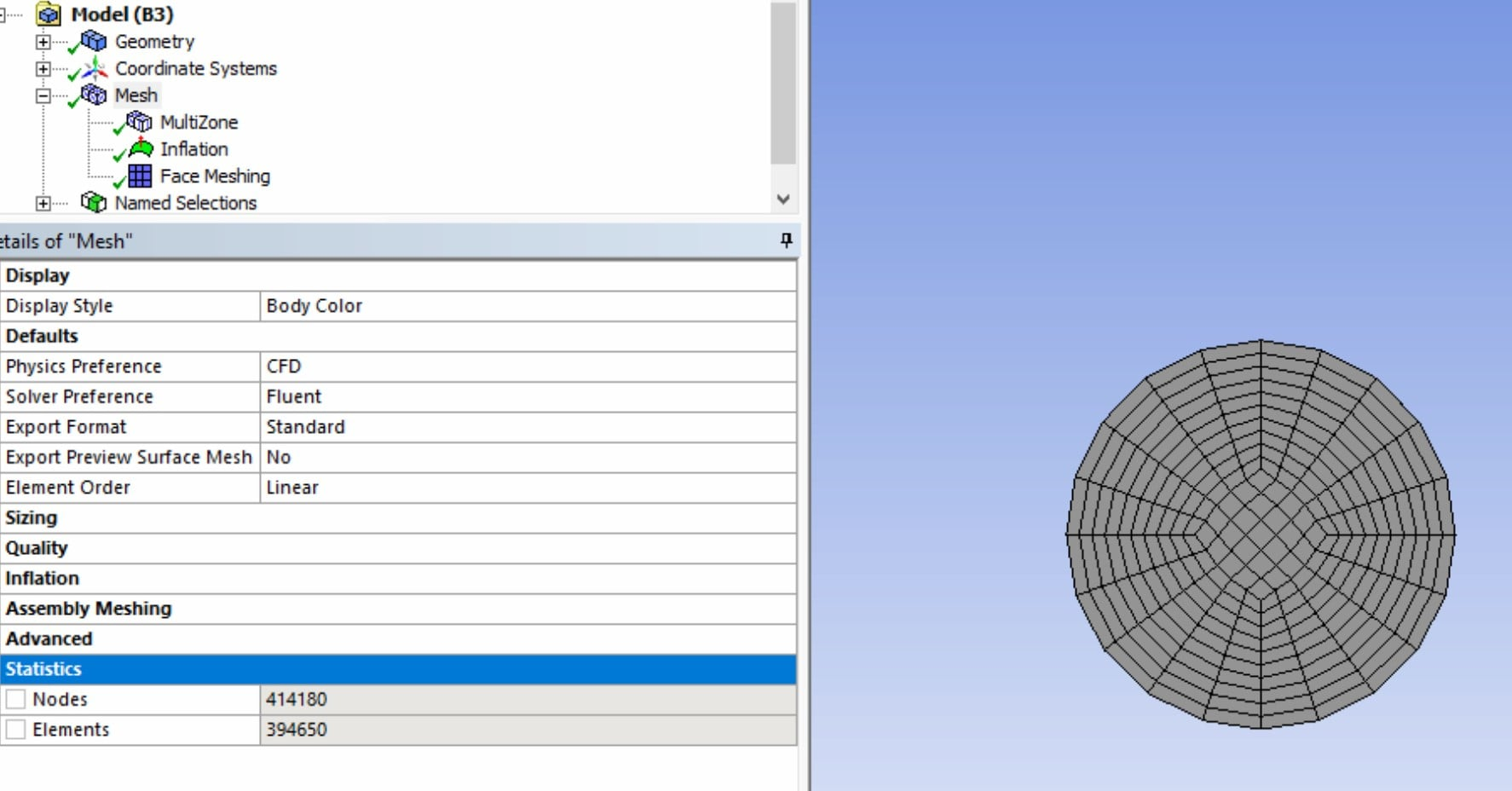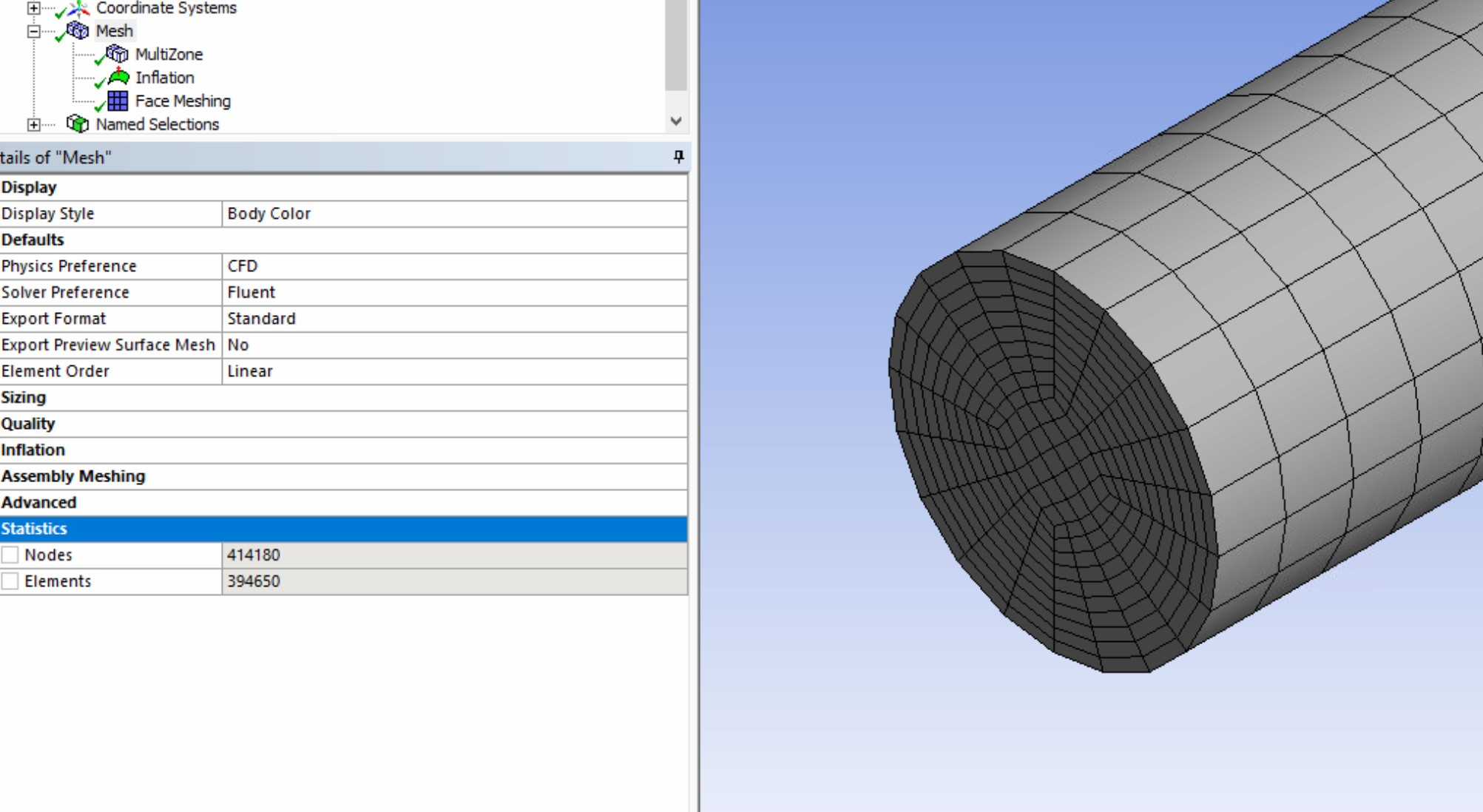• Why do you need a 3D model? What boundary conditions do you have that are not axisymmetric?

Is the flow laminar or turbulent?  Nusselt Number is all about the boundary layer. Your mesh does not have small enough element thickness at the wall.Have you divided the pipe into an entrance length where the velocity profile is developing and a downstream length where the flow is fully developed?  Are you computing the heat transfer coefficient in the fully developed region, or including the entrance length?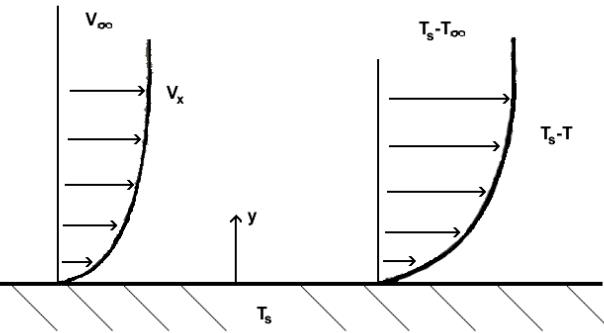• edited August 2019

My thesis advisor said my model must be in 3D

My flow is laminar and for this case my pipe diameter = 5e-5m.

Find attached more details on my mesh, acquired velocity and temperature profiles, and how I am calculating nusselt number.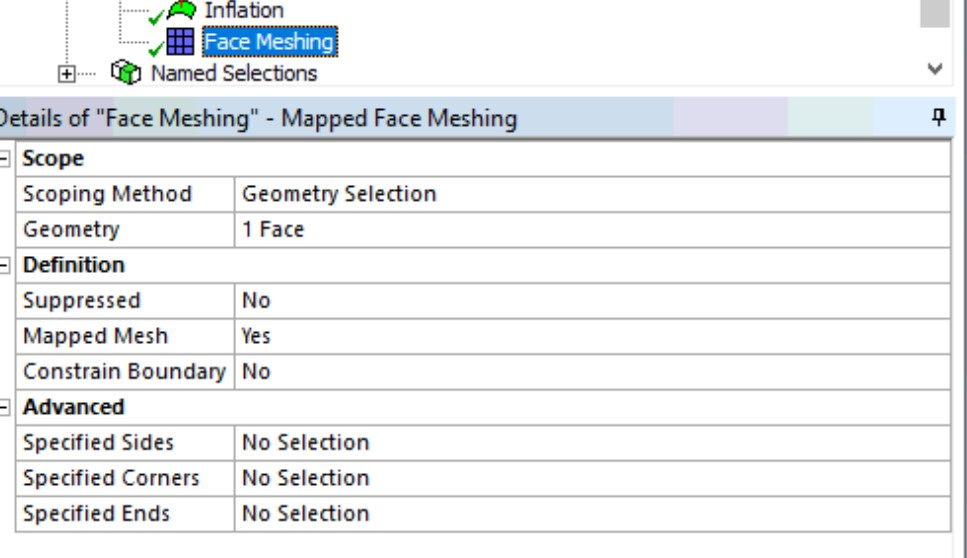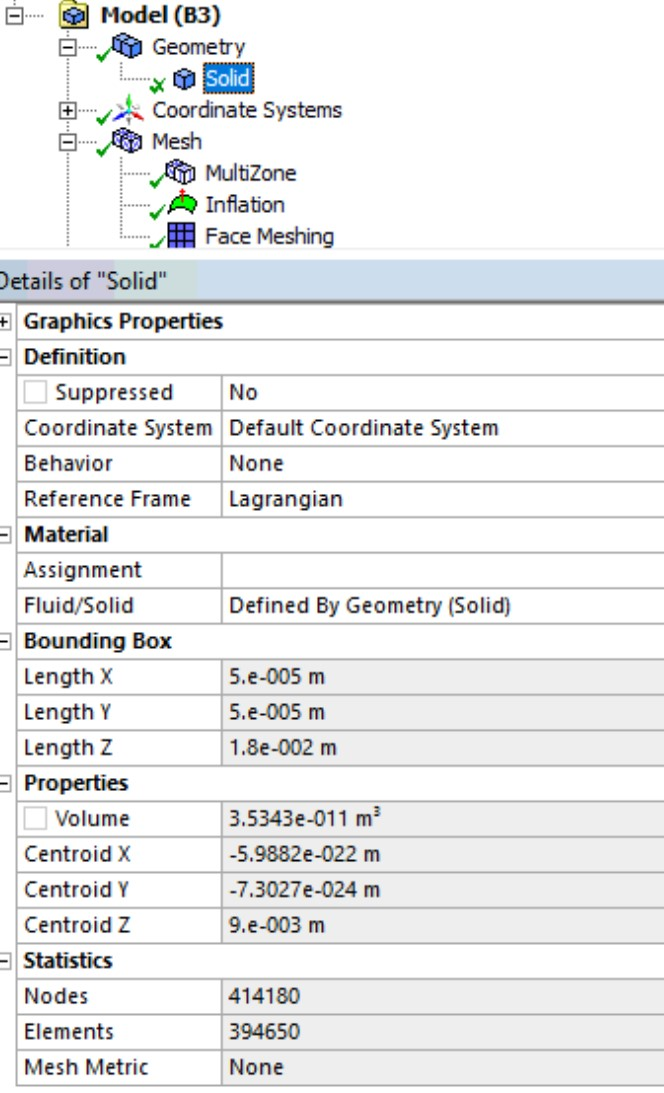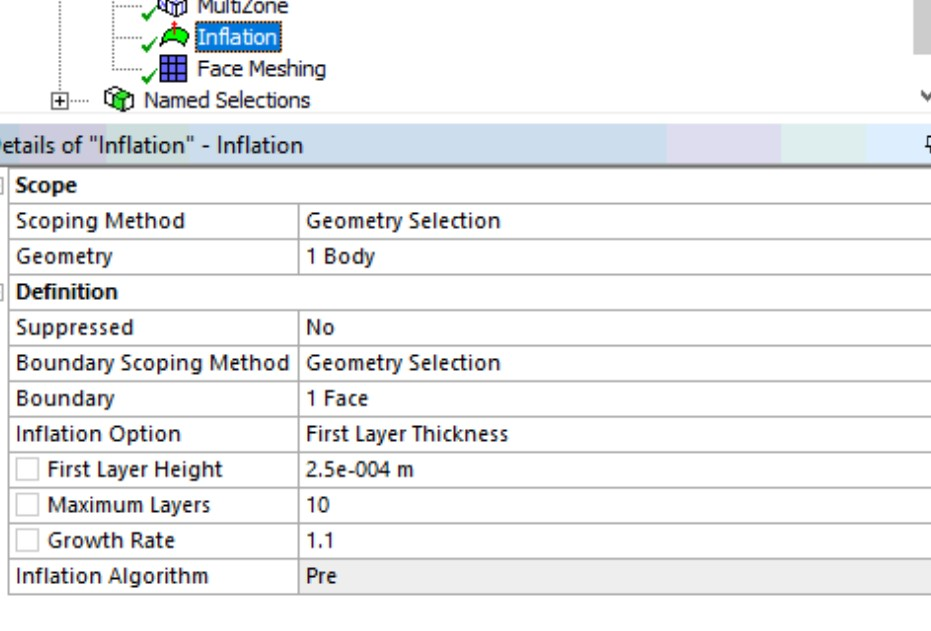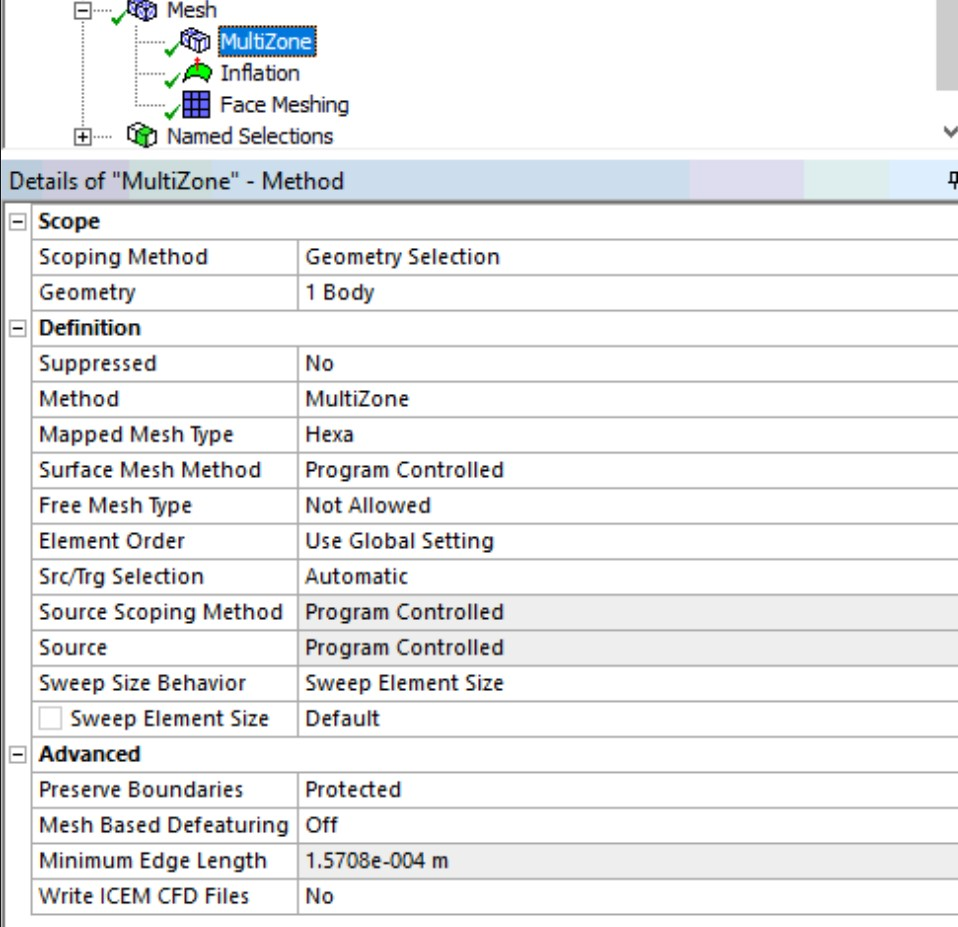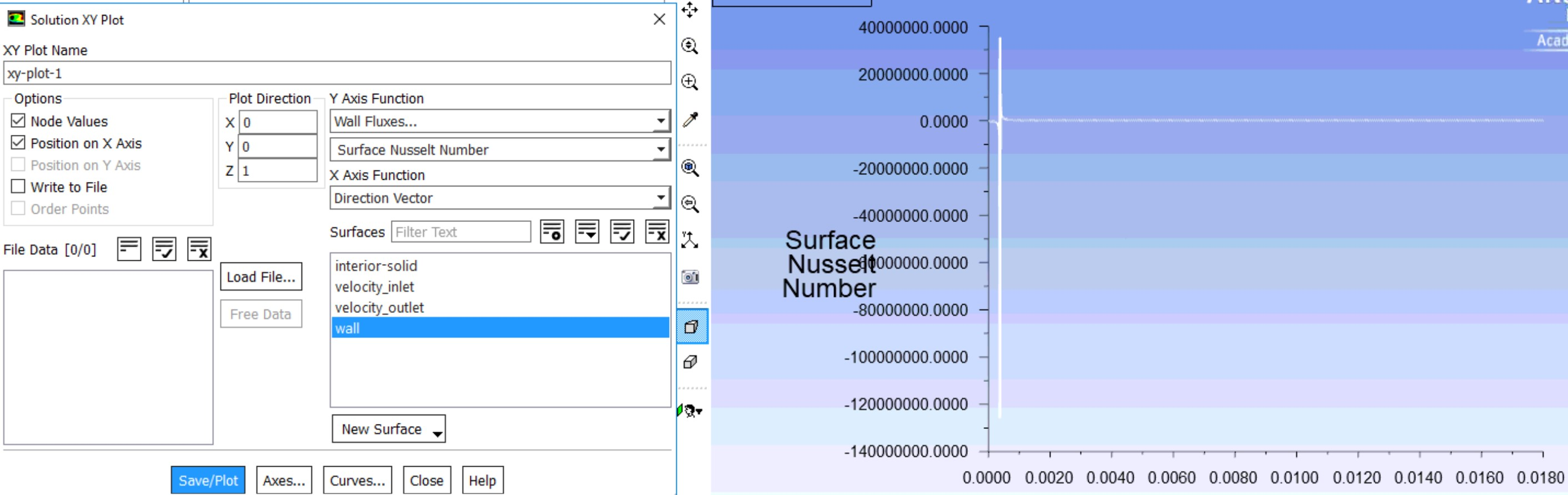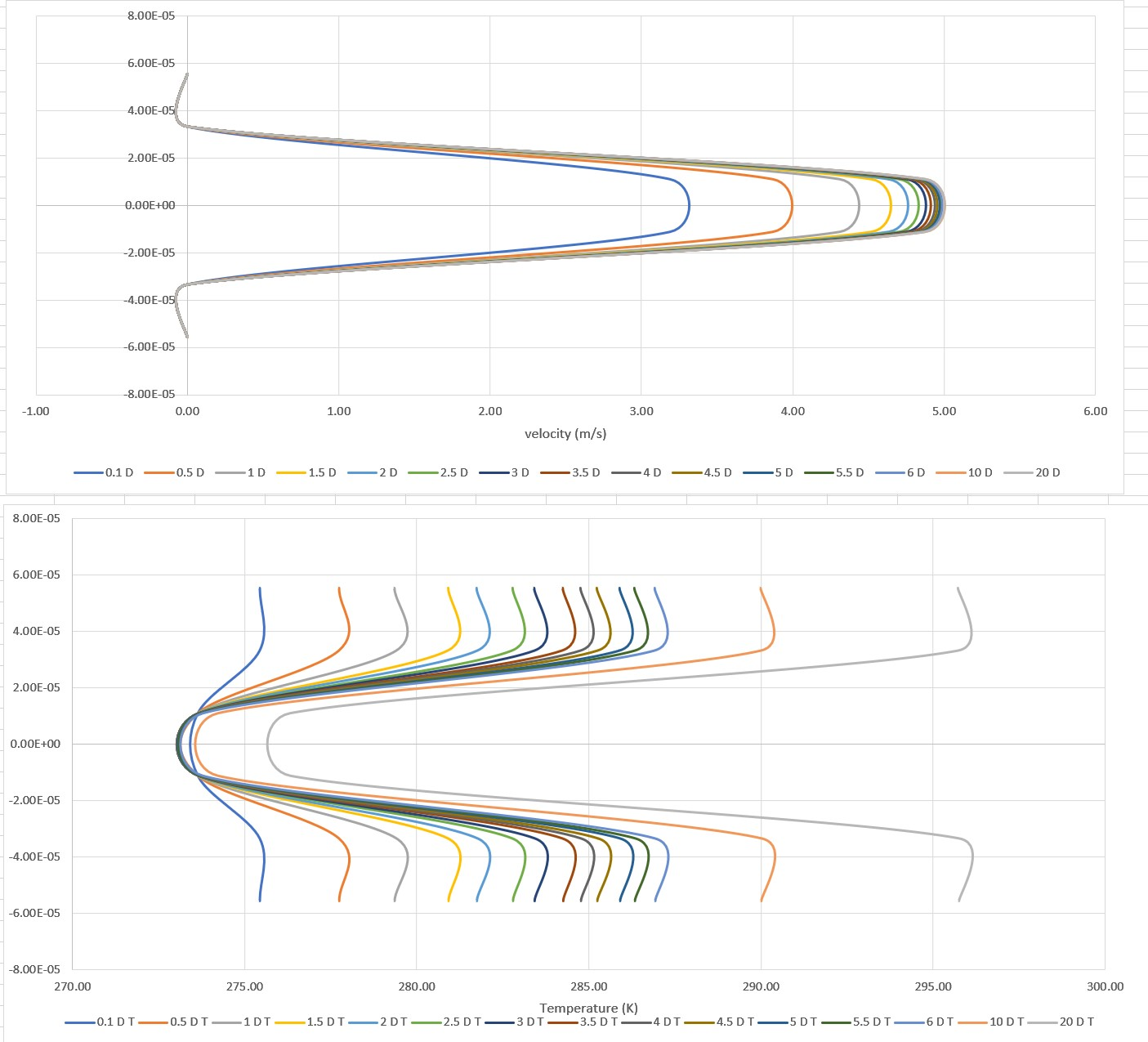• The three plots you show have no labels on either the x or y axis. I can't follow how you are calculating Nusselt number.

Have you divided the pipe into an entrance length where the velocity profile is developing and a downstream length where the flow is fully developed?  Are you computing the heat transfer coefficient in the fully developed region, or including the entrance length?

I recommend you do an axisymmetric model for yourself, even if you don't show it to your advisor. What are your advisor's reasons for requiring a 3D model?  It is foolish to build a more complicated model when a simpler model will give you the same (or better) information.

• edited August 2019

the x axes are accordingly labelled as either temperature in K or velocity in m/s and the y axis for both are the radial length from the centre (these are temperature and velocity profiles for the cross sections)

and no the pipe has three named sections (velocity inlet, velocity outlet, and wall) and the HTC is including the entrance length.

from the graphs and calculations, the flow is fully developed after about 20 diameters so I will create a separate section there to measure the HTC from. However, the nusselt curve or HTC curve measures the nusselt number or HTC at each individual  axial length coordinate rather than the whole section.

• edited August 2019

The HTC is based on a reference temperature: that's in the Reference Values in Fluent. You may be better off looking at the different HTC reports: the solver will be correct (if converged) but the reference may differ making comparison difficult.

Note, 50micron tubes are very small, so you may also be missing some physics in Fluent. This also assumes your experimental rig is accurate enough at this scale.

• edited December 2019

A side question here perhaps.@peteroznewmann
If there is a periodic unit of a pipe lets say 11 mm (Not axis symmetric becuase I have a helical cut along the extrude length in the periphery of the pipe).
How then should the nusselt number be interpreted?
As the modeled pipe is only 11 mm long, of diameter 10 mm , the (x/D value is less than 10, Incropera-Dewitt Fundamentals of HMT), so there is no scope of developed flow here.
In this case, if I am observing SHTC and Nusselt number,

1) Which formula of Nusselt is being calculated by Ansys?
2) What Nusselt value means here then?

PS: I do have a BL which shows me Y+ < 2

• edited December 2019

Except the whole purpose of periodic flow means the "length" is infinite. Please keep questions to one thread: I'll lock this one to avoid confusion.